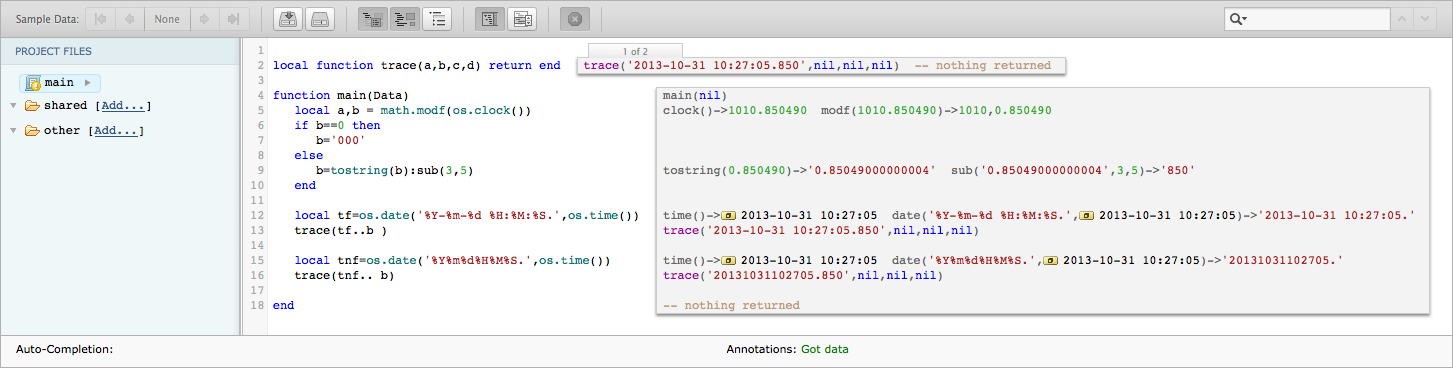# How to build milliseconds value for timestamp from os.clock() reading

Another method, to build a timestamp with milliseconds, is to use the milliseconds from the time reported by `os.clock()`.

The `os.clock()` function returns the CPU time since Lua started in seconds, with milliseconds precision.

We will use only the milliseconds part of it, and concatenate it with the current timestamp.

A small correction is needed when the milliseconds value is zero. See the example below.```function main(Data)
local a,b = math.modf(os.clock())
if b==0 then
b='000'
else
b=tostring(b):sub(3,5)
end

local tf=os.date('%Y-%m-%d %H:%M:%S.',os.time())
trace(tf..b )

local tnf=os.date('%Y%m%d%H%M%S.',os.time())
trace(tnf.. b)

end```

1.Mark Brighton

This will give a timestamp that will be unique (assuming you’re not calling it more than once a millisecond, which would be a feat), but it’s not guaranteed to be sequential, right?

In that you could call it twice in a second and get t1 > t2, if the millisecond part of the counter rolls over.

2.Julian Muir

Yes Mark that is correct.

The clock() and time() functions are not synchronized so the clock() can (and almost certainly will) rollover at sometime during a “time() second”.

As far as I am aware there is no easy method to calculate the offset, though you could loop the code for a few seconds on Iguana startup to find the min/max millisecond values and calculate an offset to zero them (if you could get the loop to run once per millisecond it would be pretty accurate).